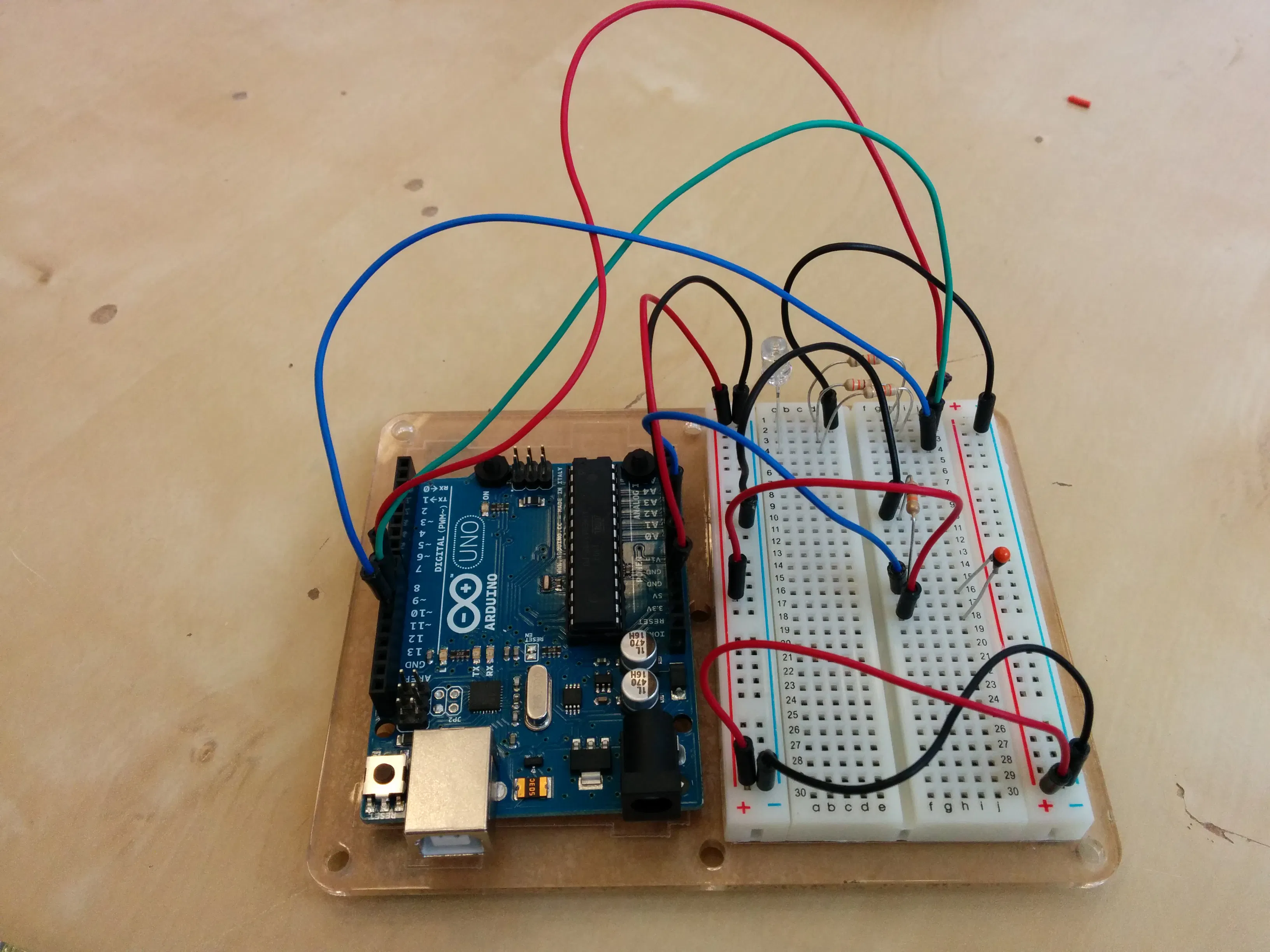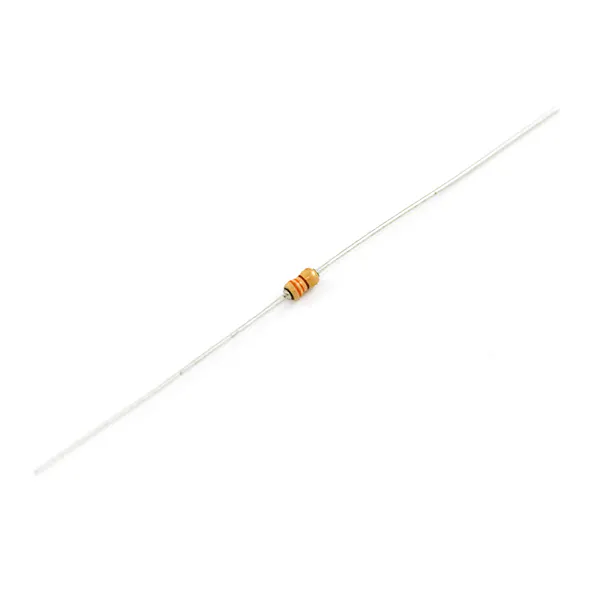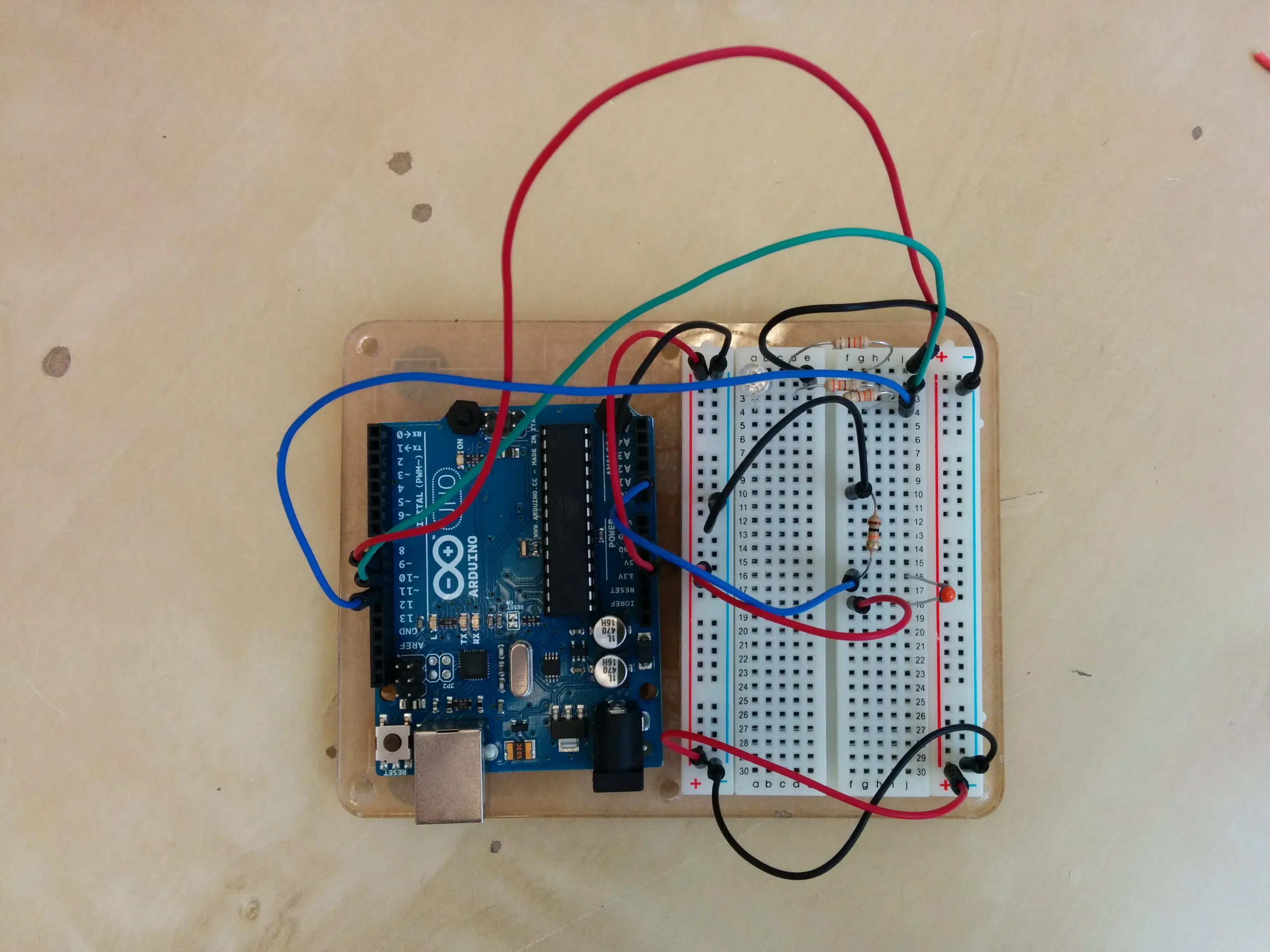Published

# Temperature-controlled RGB LED

A simple Arduino assembly that checks temperature and sets an RGB LED from blue to red.

BeginnerProtip27,642## Things used in this project

### Hardware componentsArduino UNO & Genuino UNO
×1×1Jumper wires (generic)
×11
 Thermistor
×1Resistor 10k ohm
×1Resistor 330 ohm
×3

## Schematics

### Wiring## Code

### sketch.c

C/C++
sketch.c
```#define TEMP_PIN A0
#define RED_PIN 9
#define GREEN_PIN 10
#define BLUE_PIN 11

int blue = 0, red = 0;

double resistance = ((1024.0/adc) - 1);    //calculate from voltage divider, for 10k resistor
double Temp = log(resistance);

// calculate the temperature, in K, using 4 thermistor model/material specific parameters A, B, C, D
// here we use the values for the Sparkfun/Hactronics version of the Vishay 10k NTC thermistor
Temp = 1 / (0.003354016 + 0.0002569850 * Temp + 0.000002620131 * Temp * Temp + 0.00000006383091 * Temp * Temp * Temp);
Temp = Temp - 273.15;            // Convert Kelvin to Celsius
return Temp;
}

void setLED(int blue, int red){
analogWrite(BLUE_PIN, blue);
analogWrite(RED_PIN, red);
}

void setup(){
Serial.begin(9600);
pinMode(BLUE_PIN, OUTPUT);
pinMode(RED_PIN, OUTPUT);
pinMode(GREEN_PIN, OUTPUT);
pinMode(TEMP_PIN, INPUT);
}

void loop(){
Serial.println(temp);

red = map(temp, 20, 40, 0, 255);
blue = 255 - red;

setLED(blue, red);
}
```

## Credits

### Benjamin Larralde

2 projects • 103 followers
Founder @hackster.io, hardware and crowdfunding enthusiast